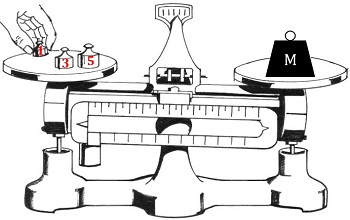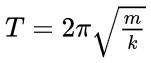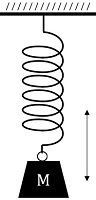# Gravitational vs. Inertial Mass: Physics Lab

Instructor: Matthew Bergstresser

Matthew has a Master of Arts degree in Physics Education. He has taught high school chemistry and physics for 14 years.

An object's gravitational mass and inertial mass are the same value, but there are different ways to determine its mass. In this lesson, we will conduct two experiments to determine the gravitational and inertial mass of an object, and compare the results.

## Which Way to Go?

Have you ever moved to a new town or gone on vacation where you do not know the area? It takes a while to get used to how to get around. One day you take one route and end up at the grocery store, and the next day you take a different route and end up at the same place! This is a similar idea to gravitational mass and inertial mass. They are two different ways to express the same thing, but measuring them requires different methods, just like driving to the same location using different routes.

Let's do two different experiments using the same mass to show that gravitational mass and inertial mass are the same thing.

## Gravitational Mass

Gravitational mass is the mass of an object based on it being measured in a gravitational field. Our first experiment will require a gravitational field, a scale, and various known masses. Getting a scale, and various known masses is not hard, and we have an excellent gravitational field here on Earth! Now, let's get down to business measuring the mass of this object. We'll start with an objective.

#### Experiment 1

Objective: To determine the mass of the object using a scale.

Procedure:

1. Acquire a balance and calibrate it.
2. Put object of unknown mass on right side of the scale.
3. Incrementally add individual known masses to the other side of scale until the arrow in the middle of the scale points at zero.

Setup and data:Analysis of data:

Notice in Diagram 1, the scale is not at equilibrium (pointing at zero). When that hand put the last mass on, the needle pointed to zero, which means the total mass on the left (9 grams) equals the mass of the object on the right. That's it. Experiment 1 is complete. We used the gravitational field of Earth along with a balance to determine the object's mass.

Conclusion: The object's mass is 9 grams.

## Inertial Mass

Determining an object's inertial mass is a little more complicated than determining its gravitational mass. Inertia is the resistance of an object to a change in its velocity. Think of a giant boulder that has been at rest in the same location for millions of years. It has quite a bit of inertia because it would take a very large force to change its velocity from zero to a non-zero value.Inertial mass is the mass of an object by applying a force to it and interpreting the results with equations. Let's do our second experiment with the same object we used in experiment 1, but instead of using a scale, we will use a spring with a known spring constant and allow the mass to oscillate on the spring. A spring's spring constant is the force required to stretch the spring a specific distance.

#### Experiment 2

Objective: To determine the inertial mass of an object using a spring.

Procedure:

1. Acquire a spring with a spring constant of 1 N/m.
2. Hang the spring, attach the object, pull the object down a few centimeters, let go, and measure how long it takes for 10 oscillations.
3. Use Equation 1 to calculate the inertial mass of the object.• T is the period of oscillation (how long it takes to go from where it starts, through its cycle, and end up back where it started). Measured in seconds (s).
• m is the mass of the object in kilograms.
• k is the spring constant in newtons-per-meter (N/m).

Setup and Data:The time required for 10 oscillations is 5.95 seconds.

Calculations and Analysis:

To unlock this lesson you must be a Study.com Member.

### Register to view this lesson

Are you a student or a teacher?